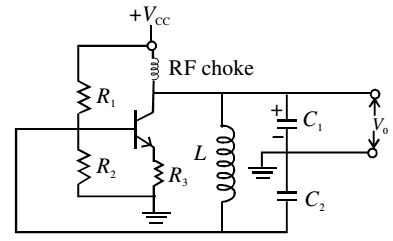# Transistor as an Oscillator

An electronic oscillator is a device which generates continuous electrical oscillations. In a simple oscillator circuit, a parallel LC circuit is used as resonant circuit and an amplifier is used to feed energy to the resonant circuit. It can generate frequencies from audio to radio range depending on the choice of L and C.

When a charged capacitor is connected across an inductor, the charge oscillates. But due to loss of energy by radiation and heating of wires, the energy is lost and the amplitude of oscillations decays with time. To build a sinusoidal oscillator, where the oscillations are sustained (i.e. they do not decay), an amplifier with positive feedback is used.

The basic idea is to feed a part of output signal in input signal. By adjusting the gain of the circuit and the phase of the feedback signal, energy dissipated in each cycle is replenished to get sustained oscillations of desired frequency.

Schematically, an oscillator is made up of two main blocks: an amplifier with gain A, and a feedback circuit with feedback factor β.In case, Aβ < 1, VO decreases continuously. On the other hand, if Aβ > 1, VO increases gradually. But if Aβ= 1, there is constant value of VO leading to sustained oscillations.

For a CE amplifier, it has 180° phase difference between the input and output, i.e. it has negative gain (–A). To keep the total feedback gain Aβ = 1, β is also negative; equal to –A–1. It is necessary to introduce a phase shift of 180° in the feedback circuit as well.In this circuit C1, C2 and L form the tank circuit. The oscillating current is generated in this circuit, which is at its resonant frequency. The output is obtained across C1, the feedback is provided across C2 connected to the base of the transistor amplifier in CE mode.

In this case 180° is introduced by the amplifier and another 180° phase shift is provided by the capacitor C2 which is connected between ground and other end of the inductor coil. Hence, the total loop gain is positive.

When the gain of transistor amplifier is sufficiently large at the resonant frequency, we obtain sustained oscillations at the output.